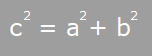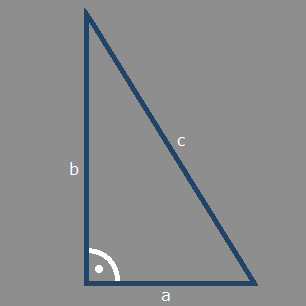What is the Pythagorean Theorem?

The Theorem applies to right triangles only. Given two sides of a right triangle you are able to calculate the length of the third side.

The Theorem says that the opposite side of the right angle (hypotenuse) squared is the sum of the other squared sides. Here is the formula:You may click on the formula to see other evaluations of the equation.

What will this site do for you?

You will be able to calculate triangle sides of right triangles with the Pythagorean Theorem.

You may enter positive floating point numbers. Any other input is considered as missing. No evaluation will take place then.

If the hypotenuse (c) is provided you have to make sure it is the longest side. If the hypotenuse is shorter than the second provided triangle side the third side is "Not a Number" (NaN).

Due to the Pythagorean Theorem you have to provide at least 2 triangle sides to calculate the third one.

After you entered 2 sides of the triangle click on "Calculate".

If you have a problem to match the triangle shape to correctly assign the sides you may click on the triangle to rotate it in 90 degrees.
Pythagorean Theorem

Calculates the sides of a right trianglea b c